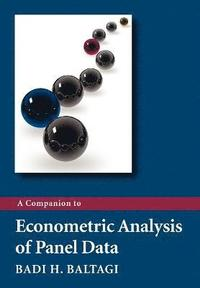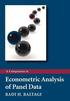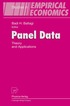Fler böcker inom
Format
Språk
Engelska
Antal sidor
308
Utgivningsdatum
2009-03-06
Upplaga
1
Förlag
John Wiley & Sons Inc
Medarbetare
Baltagi
Illustrationer
Illustrations
Dimensioner
247 x 165 x 19 mm
Vikt
526 g
Antal komponenter
1
Komponenter
67:B&W 6.69 x 9.61 in or 244 x 170 mm (Pinched Crown) Perfect Bound on White w/Gloss Lam
ISBN
9780470744031

### Du kanske gillar# A Companion to Econometric Analysis of Panel Data

559
Skickas inom 5-8 vardagar.
Fri frakt inom Sverige för privatpersoner.
This book is a companion to Baltagi's (2008) leading graduate econometrics textbook on panel data entitled Econometric Analysis of Panel Data, 4 th Edition. The book guides the student of panel data econometrics by solving exercises in a logical and pedagogical manner, helping the reader understand, learn and apply panel data methods. It is also a helpful tool for those who like to learn by solving exercises and running software to replicate empirical studies. It works as a complementary study guide to Baltagi (2008) and also as a stand alone book that builds up the reader's confidence in working out difficult exercises in panel data econometrics and applying these methods to empirical work. The exercises start by providing some background information on partitioned regressions and the Frisch-Waugh-Lovell theorem. Then it goes through the basic material on fixed and random effects models in a one-way and two-way error components models: basic estimation, test of hypotheses and prediction. This include maximum likelihood estimation, testing for poolability of the data, testing for the significance of individual and time effects, as well as Hausman's test for correlated effects. It also provides extensions of panel data techniques to serial correlation, spatial correlation, heteroskedasticity, seemingly unrelated regressions, simultaneous equations, dynamic panel models, incomplete panels, measurement error, count panels, rotating panels, limited dependent variables, and non-stationary panels. The book provides several empirical examples that are useful to applied researchers, illustrating them using Stata and EViews showing the reader how to replicate these studies. The data sets are provided on the Wiley web site: www.wileyeurope.com/college/baltagi .

## Passar bra ihop

1.2. +
3.De som köpt den här boken har ofta också köpt Panel Data av Badi H Baltagi (inbunden).

## Kundrecensioner

Har du läst boken? Sätt ditt betyg »

## Övrig information

Badi H. Baltagi is Distinguished Professor of Economics, and Senior Research Associate at the Center for Policy Research, Syracuse University. He is a fellow of the Journal of Econometrics, a recipient of the Multa and Plura Scripsit Awards from Econometric Theory, and the Journal of Applied Econometrics Distinguished Authors Award.

## Innehållsförteckning

Preface. 1 Partitioned Regression and the Frisch-Waugh-Lovell Theorem. Exercises. 1.1 Partitioned regression. 1.2 The Frisch-Waugh-Lovell theorem. 1.3 Residualing the constant. 1.4 Adding a dummy variable for the i th observation. 1.5 Computing forecasts and forecast standard errors. 2 The One-way Error Component Model. 2.1 The One-way Fixed Effects Model. Exercises. 2.1 One-way fixed effects regression. 2.2 OLS and GLS for fixed effects. 2.3 Testing for fixed effects. 2.2 The One-way Random Effects Model. Exercises. 2.4 Variance-covariance matrix of the one-way random effects model. 2.5 Fuller and Battese (1973) transformation for the one-way random effects model. 2.6 Unbiased estimates of the variance components: the one-way model. 2.7 Feasible unbiased estimates of the variance components: the one-way model. 2.8 Gasoline demand in the OECD. 2.9 System estimation of the one-way model: OLS versus GLS. 2.10 GLS is a matrix weighted average of between and within. 2.11 Efficiency of GLS compared to within and between estimators. 2.12 Maximum likelihood estimation of the random effects model. 2.13 Prediction in the one-way random effects model. 2.14 Mincer wage equation. 2.15 Bounds for s 2 in a one-way random effects model. 2.16 Heteroskedastic fixed effects models. 3 The Two-way Error Component Model. 3.1 The Two-way Fixed Effects Model. Exercise. 3.1 Two-way fixed effects regression. 3.2 The Two-way Random Effects Model. Exercises. 3.2 Variance-covariance matrix of the two-way random effects model. 3.3 Fuller and Battese (1973) transformation for the two-way random effects model. 3.4 Unbiased estimates of the variance components: the two-way model. 3.5 Feasible unbiased estimates of the variance components: the two-way model. 3.6 System estimation of the two-way model: OLS versus GLS. 3.7 Prediction in the two-way random effects model. 3.8 Variance component estimation under misspecification. 3.9 Bounds for s 2 , in a two-way random effects model. 3.10 Nested effects. 3.11 Three-way error component model. 3.12 A mixed error component model. 3.13 Productivity of public capital in private production. 4 Test of Hypotheses Using Panel Data. 4.1 Tests for Poolability of the Data. Exercises. 4.1 Chow (1960) test. 4.2 Roy (1957) and Zellner (1962) test. 4.2 Tests for Individual and Time Effects. Exercises. 4.3 Breusch and Pagan (1980) Lagrange multiplier test. 4.4 Locally mean most powerful one-sided test. 4.5 Standardized Honda (1985) test. 4.6 Standardized King and Wu (1997) test. 4.7 Conditional Lagrange multiplier test: random individual effects. 4.8 Conditional Lagrange multiplier test: random time effects. 4.9 Testing for poolability using Grunfeld's data. 4.10 Testing for random time and individual effects using Grunfeld's data. 4.3 Hausman's Test for Correlated Effects. Exercises. 4.11 Hausman (1978) test based on a contrast of two estimators. 4.12 Hausman (1978) test based on an artificial regression. 4.13 Three contrasts yield the same Hausman test. 4.14 Testing for correlated effects in panels. 4.15 Hausman's test as a Gauss-Newton regression. 4.16 Hausman's test using Grunfeld's data. 4.17 Relative efficiency of the between estimator with respect to the within estimator. 4.18 Hausman's test using Munnell's data. 4.19 Currency Union and Trade. 5 Heteroskedasticity and Serial Correlation. 5.1 Heteroskedastic Error Component Model. Exercises. 5.1 Heteroskedastic individual effects. 5.2 An alternative heteroskedastic error component model. 5.3 An LM test for heteroskedasticity in a one-way error component model. 5.2 Serial Correlation in the Error Component Model. Exercises. 5.4 AR(1) process. 5.5 Unbiased estimates of the variance components under the AR(1) model. 5.6 AR(2) process. 5.7 AR(4) process for quarterly data. 5.8 MA(1) process. 5.9 MA( q ) process. 5.10 Prediction in the serially correlated error component model. 5.11 A joint LM test for serial correlation and random individual